Worksheets and No Prep Teaching Resources
Math Worksheets

# Left and Right, Above and Below Worksheets

Understanding left and right shouldn't be left to chance, and these exciting worksheets will give students the right ideas to thoroughly ground them in this important topic. These sensational worksheets cover upper and lower-case letters and numbers in a variety of attractive formats that will be both fun and appealing for students. Other creative worksheets include fill-ins and multiple choice, so students are never bored as they learn this necessary concept that carries over into so many subjects.

Writing Uppercase Letters to the Left and Right
Writing Uppercase Letters to the Left and Right - Easier: Pictorial Directions Given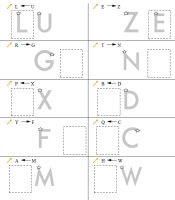Left and Right: Trace Hint On Each - First Two Trace Answers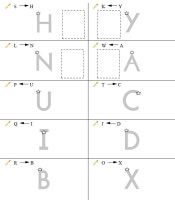Left and Right: Trace Hint On First 4 Problems - First Two Trace Answers Left and Right: Trace Hint On First 4 Problems

Writing Uppercase Letters to the Left and Right - Pictorial And English Directions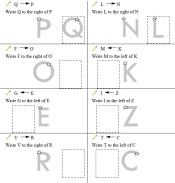Left and Right: Trace Hint On Each - First Two Trace Answers Left and Right: Trace Hint On First 4 Problems - First Two Trace Answers Left and Right: Trace Hint On First 4 Problems

Writing Uppercase Letters to the Left and Right - Pictorial Directions for First Two Problems then English Directions Only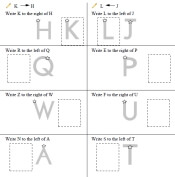Left and Right: Trace Hint On Each - First Two Trace Answers Left and Right: Trace Hint On First 4 Problems - First Two Trace Answers Left and Right: Trace Hint On First 4 Problems

Writing Uppercase Letters to the Left and Right - English Directions Only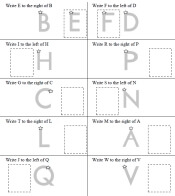Left and Right: Trace Hint On Each - First Two Trace Answers Left and Right: Trace Hint On First 4 Problems - First Two Trace Answers Left and Right: Trace Hint On First 4 Problems

Writing Numbers to the Left and Right
Writing Numbers to the Left and Right - Easier: Pictorial Directions Given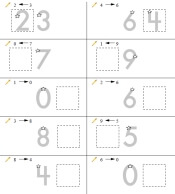Left and Right: Trace Hint On Each - First Two Trace Answers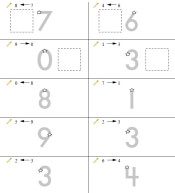Left and Right: Trace Hint On First 4 Problems - First Two Trace Answers Left and Right: Trace Hint On First 4 Problems

Writing Numbers to the Left and Right - Pictorial And English Directions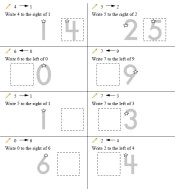Left and Right: Trace Hint On Each - First Two Trace Answers Left and Right: Trace Hint On First 4 Problems - First Two Trace Answers Left and Right: Trace Hint On First 4 Problems

Writing Numbers to the Left and Right - Pictorial Directions for First Two Problems then English Directions Only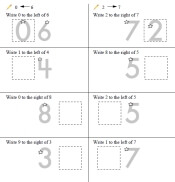Left and Right: Trace Hint On Each - First Two Trace Answers Left and Right: Trace Hint On First 4 Problems - First Two Trace Answers Left and Right: Trace Hint On First 4 Problems

Writing Numbers to the Left and Right - English Directions Only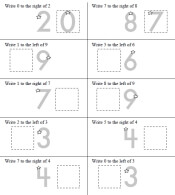Left and Right: Trace Hint On Each - First Two Trace Answers Left and Right: Trace Hint On First 4 Problems - First Two Trace Answers Left and Right: Trace Hint On First 4 Problems

Writing Numbers to the Left, Right, Above, and Below
Writing Numbers to the Left, Right, Above, and Below - Easier: Pictorial Directions Given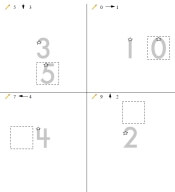Left, Right, Above, and Below: Trace Hint On Each - First Two Trace Answers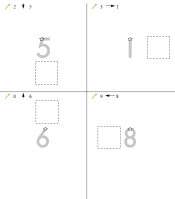Left, Right, Above, and Below: Trace Hint On First 4 Problems - First Two Trace Answers Left, Right, Above, and Below: Trace Hint On First 4 Problems

Writing Numbers to the Left, Right, Above, and Below - Pictorial And English Directions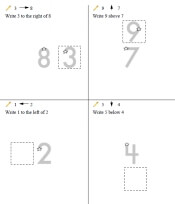Left, Right, Above, and Below: Trace Hint On Each - First Two Trace Answers Left, Right, Above, and Below: Trace Hint On First 4 Problems - First Two Trace Answers Left, Right, Above, and Below: Trace Hint On First 4 Problems

Writing Numbers to the Left, Right, Above, and Below - Pictorial Directions for First Two Problems then English Directions Only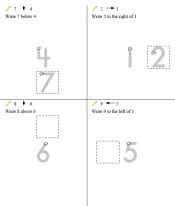Left, Right, Above, and Below: Trace Hint On Each - First Two Trace Answers Left, Right, Above, and Below: Trace Hint On First 4 Problems - First Two Trace Answers Left, Right, Above, and Below: Trace Hint On First 4 Problems

Writing Numbers to the Left, Right, Above, and Below - English Directions Only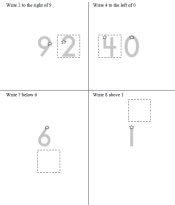Left, Right, Above, and Below: Trace Hint On Each - First Two Trace Answers Left, Right, Above, and Below: Trace Hint On First 4 Problems - First Two Trace Answers Left, Right, Above, and Below: Trace Hint On First 4 Problems

Locations: Right, Left, Below, and Above
Easier - Help on Top and Larger Images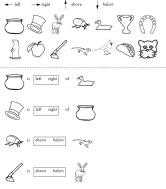Multiple Choice: Left and Right Multiple Choice: Above and Below Multiple Choice: Left, Right, Above and Below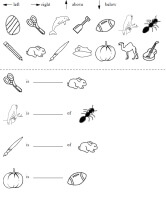Fill-in: Left and Right Fill-in: Above and Below Fill-in: Left, Right, Above and Below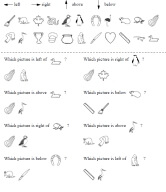Picture Multiple Choice: Left and Right Picture Multiple Choice: Above and Below Picture Multiple Choice: Left, Right, Above and Below

Harder - No Help on Top and Smaller Images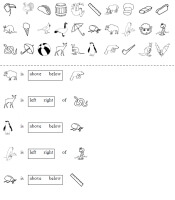Multiple Choice: Left and Right Multiple Choice: Above and Below Multiple Choice: Left, Right, Above and Below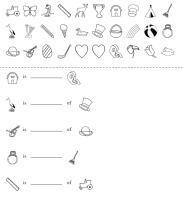Fill-in: Left and Right Fill-in: Above and Below Fill-in: Left, Right, Above and Below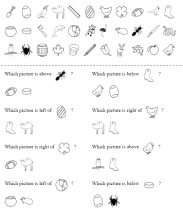Picture Multiple Choice: Left and Right Picture Multiple Choice: Above and Below Picture Multiple Choice: Left, Right, Above and Below

Following Directions - Locations: Right, Left, Below, and Above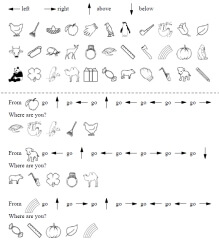Following Directions

Kindergarten Geometry - Introduction to Drawing Some Simple Polygons

Ordered Pairs Printables

Have a suggestion or would like to leave feedback?